# 12 4 Quadratic Functions Goal Graph Quadratic functions

• Slides: 13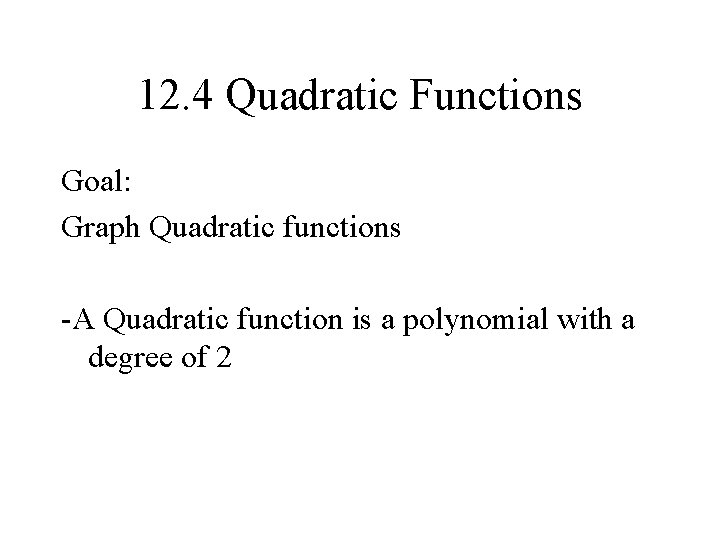12. 4 Quadratic Functions Goal: Graph Quadratic functions -A Quadratic function is a polynomial with a degree of 2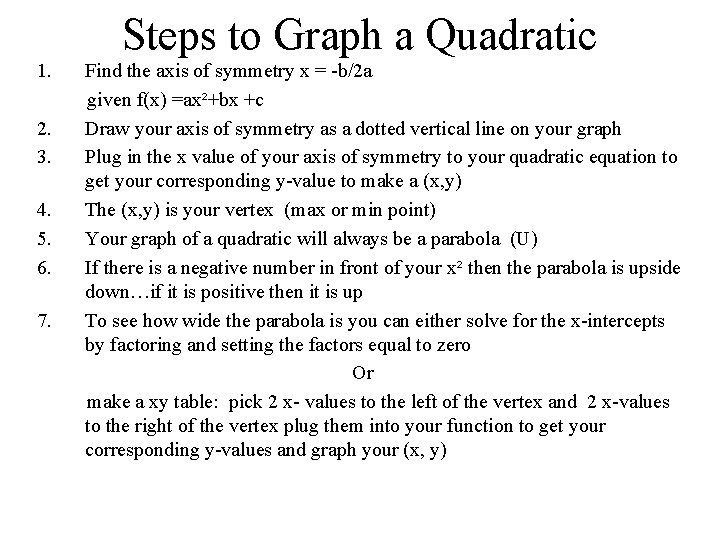Steps to Graph a Quadratic 1. 2. 3. 4. 5. 6. 7. Find the axis of symmetry x = -b/2 a given f(x) =ax²+bx +c Draw your axis of symmetry as a dotted vertical line on your graph Plug in the x value of your axis of symmetry to your quadratic equation to get your corresponding y-value to make a (x, y) The (x, y) is your vertex (max or min point) Your graph of a quadratic will always be a parabola (U) If there is a negative number in front of your x² then the parabola is upside down…if it is positive then it is up To see how wide the parabola is you can either solve for the x-intercepts by factoring and setting the factors equal to zero Or make a xy table: pick 2 x- values to the left of the vertex and 2 x-values to the right of the vertex plug them into your function to get your corresponding y-values and graph your (x, y)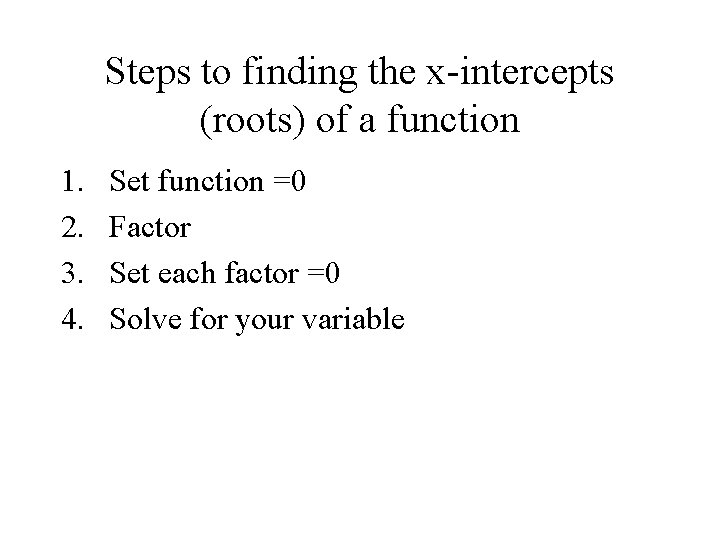Steps to finding the x-intercepts (roots) of a function 1. 2. 3. 4. Set function =0 Factor Set each factor =0 Solve for your variableFind the x-intercepts of f(x) = x 2 – 5 x + 6 Set the function = zero and solve.Model Graph Axis of Symmetry Parabola VertexModel graph Axis of Symmetry Vertex Parabola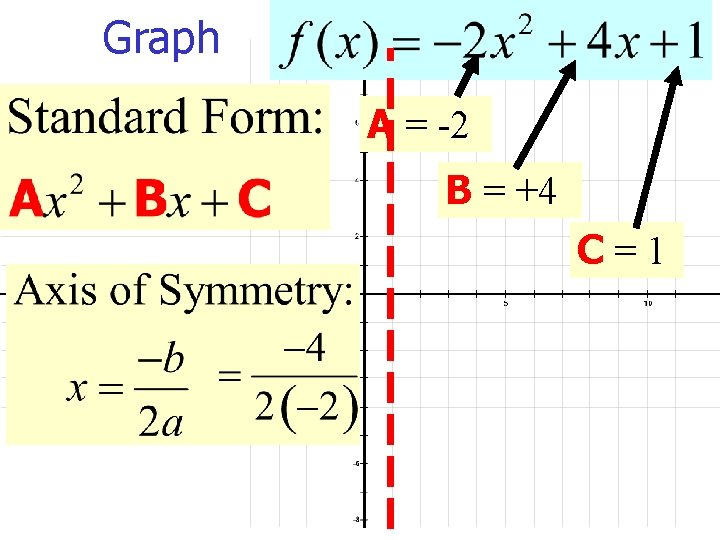Graph A = -2 B = +4 C=1Graph x 3 2 1 0 -1 y -5 1 3 1 -5 Axis of symmetry is x= 1 Vertex: y= -2(1)² + 4(1) +1 y= -2 +4+1 y=3 Vertex : (1, 3) X-intercepts 0 = -2 x² +4 +1 0= not factorable as of now so use a xy chart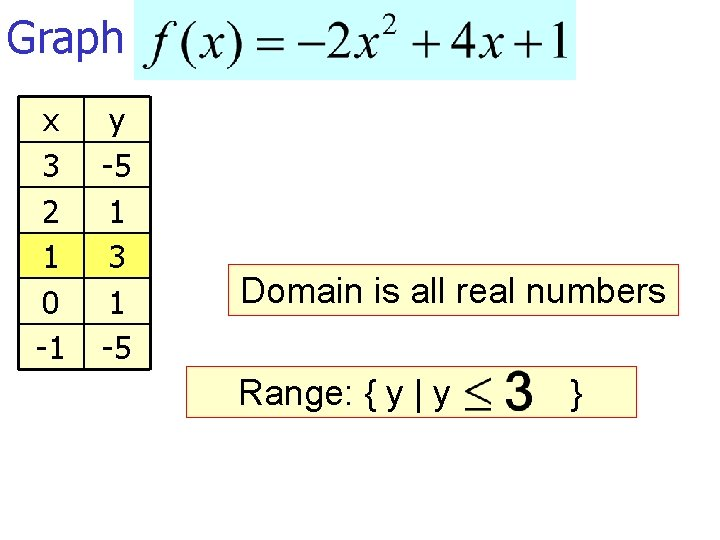Graph x 3 2 1 0 -1 y -5 1 3 1 -5 Domain is all real numbers Range: { y | y }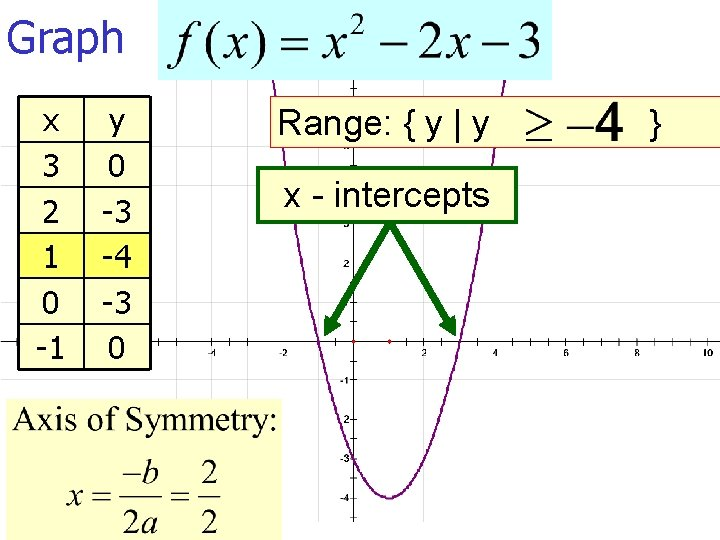Graph x 3 2 1 0 -1 y 0 -3 -4 -3 0 Range: { y | y x - intercepts }x y 2 0 0 4 -1 4. 5 -2 4 -4 0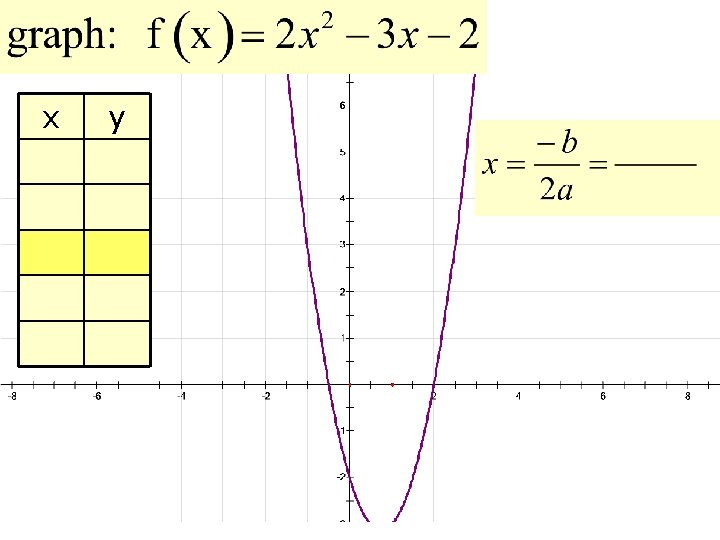x y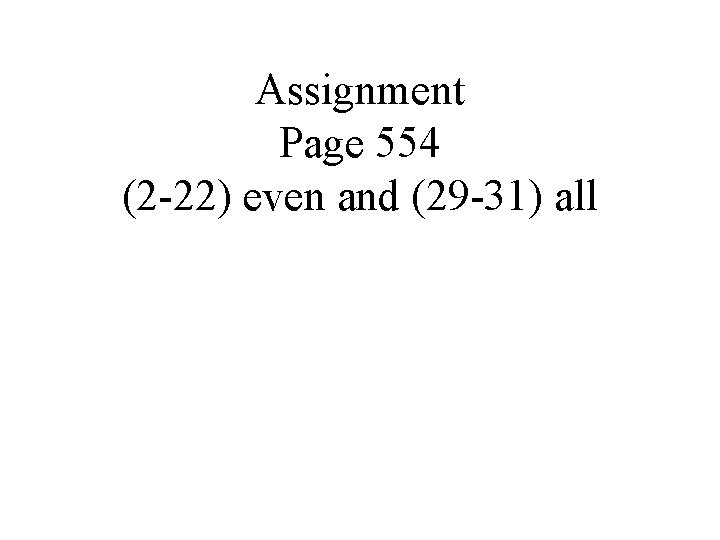Assignment Page 554 (2 -22) even and (29 -31) all